## Here Global users can buy Chinese products with ease from 1688,Taobao,Tmall.

Paste the product link (URL) below, one product URL at a time

### Do You Need Help?

•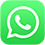WhatsApp Me
•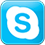Skype:doesbuy
•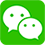Wechat:mwkk1688
•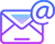service@doesbuy.com

## How to Calculate Chargeable Weight for Air Freight？

Calculator tools:

Conversion tool: http://cargotracking.utopiax.org/convert2.html

Volumetric Weight calculator: http://www.online-calculators.co.uk/volumetric/weight.php

The Chargeable Weight of Air Freight shipments are calculated as the Actual Weight (Gross Weight) or the Volumetric (also called Volume or Dimensional) Weight of the shipment, whichever is the greater. This uses an estimated weight that is calculated based on the dimensions (length, width and height) of a package (shipments are always shown in the order of L x W x H). Typically, large items with a light overall weight take up more space on an aircraft than a small, heavy item. That’s why the Airlines charge according to Chargeable Weight.

Chargeable weight is commonly used by air freight forwarders, domestic motor carriers and brokers to calculate their air freight and/or domestic trucking charges.

For those of you who simply want the formulas without a detailed explanation, here you go:

The formula for calculating the volume/dimensional weight for all commodities is 166 cubic inches per pound or 6000 cubic centimeters per kilogram or 366 cubic inches per kilogram.

Multiply the length by the width by the height to obtain the cubic inches, then:

• To obtain the dimensional weight in pounds using inches, divide the cubic inch result by 166
• To obtain the dimensional weight in kilograms using inches, divide the cubic inch result by 366
• Using Dimensions in Centimeters: To obtain the dimensional weight in kilograms using centimeters, divide the cubic centimeter result by 5000 or 6000

Some definitions and formulas to start: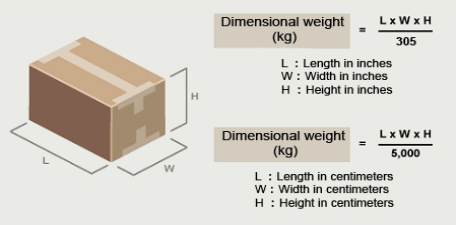Chargeable Weight: the greater of actual weight vs. volume weight of a shipment.

Chargeable weight is an equilibrium point where the actual weight and volume weight of cargo balance out for the airline, BUT, keep in mind that if the actual weight of the cargo is higher than the “equilibrium point”, the air freight charges are billed on that actual weight.

Volume/Volumetric/Dimensional Weight: Cargo weight based on dimensions of the cargo

Actual Weight: Actual weight of the cargo weighed on a scale

Lb or lbs: pounds

Kg or kgs: kilograms

Cft or ft3: cubic feet

Cbm or m3: cubic meters

Tonne or mt: metric ton 1,000 kgs / 2,204.6 lbs

Basic Conversions:

1 inch = 2.54 centimeters (cms)
1 lbs = 2.20462 kgs

Imperial shipping factor examples:

167 in3/lb = 10.4  lb/ft3

Metric shipping factor examples:

5000  cm3/kg = 200  kg/m3
6000  cm3/kg = 166.667  kg/m3
7000  cm3/kg = 142.857  kg/m3

Note: all dimensions and weights are rounded to the nearest whole number.

Combining dimensions in inches and weight in kgs: (L x W x H)/366 (this is commonly done in U.S. exports since dimensions are provided in inches but charges for air freight are always in kgs)

How do I Calculate Volumetric Weight to determine the Chargeable Weight of my Air shipment?

Example 1 – Actual Weight is Greater than Volume Weight:

ABC Company is going to ship some cargo from Shanghai to LosAngeles.   The shipment consists of 5 boxes/cartons, each weighing 30kgs, and each measuring 50 x 40 x 40 cms   / 19.685 x 15.748 x 15.748 inches.
Actual Gross Weight = 5 boxes x 30kgs each = 150 kgs
Volumetric Weight in kgs using centimeters = (50 x 40 x 40cm) x 5 boxes / 6000 = 67kgs
Volumetric Weight in kgs using inches = (20 x 16 x 16) x 5 boxes / 366 = 67kgs
Result: 150 kgs is greater than 67 kgs so the Chargeable Weight will be 150 kgs which, in this case, is the Actual Weight.

Example 2 – Volume Weight is Greater than Actual Weight:

ABC Company is going to ship some cargo from Shanghai to Los Angeles.   The shipment consists of 3 boxes/cartons, each weighing 10kgs, and each measuring 50 x 40 x 40 cms / 19.6 x 15.7 x 15.7 inches.
Actual Gross Weight = 3 boxes x 10 kgs each = 30 kgs
Volumetric Weight in kgs using centimeters = (50 x 40 x 40cm) x 3 boxes / 6000 = 40 kgs
Volumetric Weight in kgs using inches = (19.6 x 15.7 x 15.7) x 3 boxes / 366 = 40 kgs
Result: 40kgs is greater than 30kgs so the Chargeable Weight will be 40kgs which is also the Volume Weight.

Alternatively you can click here http://cargotracking.utopiax.org/convert2.html to use a conversion tool that calculates chargeable weight to determine if your shipment will be billed on chargeable weight or actual weight.

How does DHL/Fedex/UPS/TNT/Canada Post calculate Air Freight Charges?

Centimeters / Kilograms:

DHL: (L cm A— W cm A— H cm)/5000 or 4000  depending on certain import/country criteria
FedEx: (L cm A— W cm A— H cm)/6000 (new) or /5000 (old, still used in Asia) for international shipments, (L cm x W cm x H cm)/7000 for domestic shipments
UPS: (L cm A— W cm A— H cm)/6000 or /5000 depending on certain import/country criteria
Inches / Pounds
FedEx Express & UPS: (L in A— W in A— H in)/139
FedEx Ground & UPS Ground: (L in A— W in A— H in)/139
FYI: The above divisors are subject to change by the carriers. Please visit their respective websites for more information.

Calculating the Volumetric Weight of a Package:

http://www.online-calculators.co.uk/volumetric/weight.php